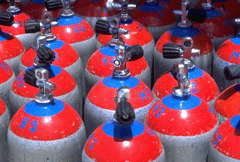Ch 1. Basics Multimedia Engineering Fluids MassDensity IdealGas Law Viscosity SurfaceTension VaporPressure
 Chapter 1. Basics 2. Fluid Statics 3. Kinematics 4. Laws (Integral) 5. Laws (Diff.) 6. Modeling/Similitude 7. Inviscid 8. Viscous 9. External Flow 10. Open-Channel Appendix Basic Math Units Basic Equations Water/Air Tables Sections Search eBooks Dynamics Fluids Math Mechanics Statics Thermodynamics Author(s): Chean Chin Ngo Kurt Gramoll ©Kurt GramollFLUID MECHANICS - CASE STUDY SOLUTIONCompressed Tanks

Table: Gas Constants for the Problem
 Gas Constant, R (kJ/kg-K) Air 0.2870 Ammonia 0.4882 Carbon Dioxide 0.1889 Helium 2.0771 Hydrogen 4.1243 Nitrogen 0.2968 Oxygen 0.2598 R-12 0.06876 R-134a 0.08149

The conditions of the gases are given as follows:
 Number of Tanks Temperature (K) Absolute Pressure (MPa) Oxygen 5 290 1.00 Nitrogen 4 290 0.85 Carbon Dioxide 6 290 1.25

The mass of each gas can be determined by the ideal gas law:

m = pV/RT

For oxygen, the gas constant R is 0.2598 kJ/kg-K. The mass of the oxygen in a single tank is

m = (1000)(0.25)/(0.2598)(290)
= 3.318 kg

For nitrogen, the gas constant R is 0.2968 kJ/kg-K. The mass of the nitrogen in a single tank is

m = (850)(0.25)/(0.2968)(290)
= 2.469 kg

For carbon dioxide, the gas constant R is 0.1889 kJ/kg-K. The mass of the carbon dioxide in a single tank is

m = (1,250)(0.25)/(0.1889)(290) = 5.705 kg

The total weight of the tanks is then given by

Wtotal = weight of empty tanks + weight of gases
= (15)(5)(9.8) + (5)(3.318)(9.8)
+ (4)(2.469)(9.8) + (6)(5.705)(9.8)
= 1,330 N

Practice Homework and Test problems now available in the 'Eng Fluids' mobile app
Includes over 250 problems with complete detailed solutions.
Available now at the Google Play Store and Apple App Store.